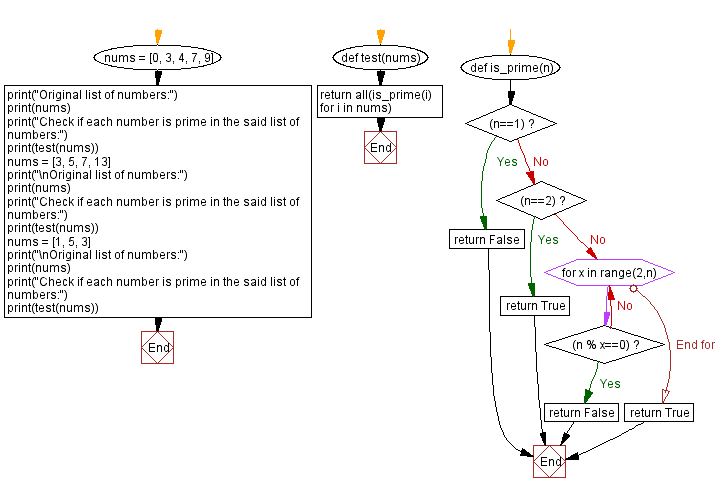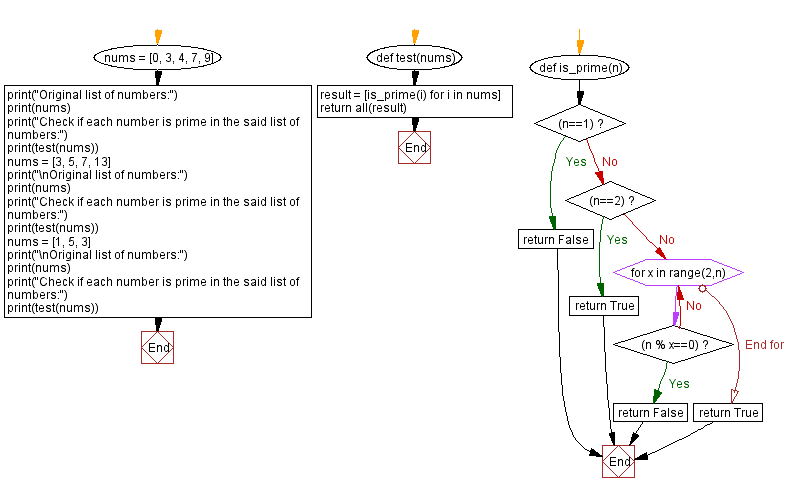﻿ Python - Check if each number is prime in a list of numbers# Python Exercises: Check if each number is prime in a list of numbers

## Python List: Exercise - 279 with Solution

Write a Python program to check if each number is prime in a given list of numbers. Return True if all numbers are prime otherwise False.

Sample Data:
([0, 3, 4, 7, 9]) -> False
([3, 5, 7, 13]) -> True
([1, 5, 3]) -> False

Sample Solution-1:

Python Code:

``````def test(nums):
return all(is_prime(i) for i in nums)
def is_prime(n):
if (n==1):
return False
elif (n==2):
return True;
else:
for x in range(2,n):
if(n % x==0):
return False
return True

nums = [0, 3, 4, 7, 9]
print("Original list of numbers:")
print(nums)
print("Check if each number is prime in the said list of numbers:")
print(test(nums))
nums = [3, 5, 7, 13]
print("\nOriginal list of numbers:")
print(nums)
print("Check if each number is prime in the said list of numbers:")
print(test(nums))
nums = [1, 5, 3]
print("\nOriginal list of numbers:")
print(nums)
print("Check if each number is prime in the said list of numbers:")
print(test(nums))
```
```

Sample Output:

```Original list of numbers:
[0, 3, 4, 7, 9]
Check if each number is prime in the said list of numbers:
False

Original list of numbers:
[3, 5, 7, 13]
Check if each number is prime in the said list of numbers:
True

Original list of numbers:
[1, 5, 3]
Check if each number is prime in the said list of numbers:
False
```

Flowchart:## Visualize Python code execution:

The following tool visualize what the computer is doing step-by-step as it executes the said program:

Sample Solution-2:

Python Code:

``````def test(nums):
result = [is_prime(i) for i in nums]
return all(result)

def is_prime(n):
if (n==1):
return False
elif (n==2):
return True;
else:
for x in range(2,n):
if(n % x==0):
return False
return True

nums = [0, 3, 4, 7, 9]
print("Original list of numbers:")
print(nums)
print("Check if each number is prime in the said list of numbers:")
print(test(nums))
nums = [3, 5, 7, 13]
print("\nOriginal list of numbers:")
print(nums)
print("Check if each number is prime in the said list of numbers:")
print(test(nums))
nums = [1, 5, 3]
print("\nOriginal list of numbers:")
print(nums)
print("Check if each number is prime in the said list of numbers:")
print(test(nums))
```
```

Sample Output:

```Original list of numbers:
[0, 3, 4, 7, 9]
Check if each number is prime in the said list of numbers:
False

Original list of numbers:
[3, 5, 7, 13]
Check if each number is prime in the said list of numbers:
True

Original list of numbers:
[1, 5, 3]
Check if each number is prime in the said list of numbers:
False
```

Flowchart:## Visualize Python code execution:

The following tool visualize what the computer is doing step-by-step as it executes the said program:

Python Code Editor:

Have another way to solve this solution? Contribute your code (and comments) through Disqus.

Previous Python Exercise: Sum of missing numbers of a list of integers.
Next Python Exercise: Extract the first n number of vowels from a string.

What is the difficulty level of this exercise?

Test your Programming skills with w3resource's quiz.

﻿

## Python: Tips of the Day

Getting sorted iterable (can sort by "compare" function):

```>>> a = [1, 2, -3]
>>> sorted(a)
[-3, 1, 2]

>>> sorted(a,key=abs)
[1, 2, -3]
```